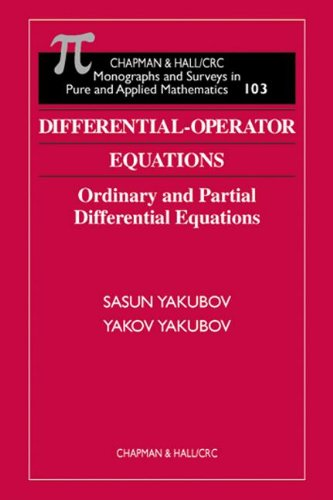# [PDF] Differential-Operator Equations: Ordinary and Partial Differential Equations (Monographs and Surveys in Pure and Applied Mathematics)

#### Category: Tutorial

Posted on 2017-12-27, by luongquocchinh.

DescriptionAuthor: Yakov Yakubov | Category: Mathematics | Language: English | Page: 576 | ISBN: 1584881399 | ISBN13: 9781584881391 |

Description: The theory of differential-operator equations is one of two modern theories for the study of both ordinary and partial differential equations, with numerous applications in mechanics and theoretical physics. Although a number of published works address differential-operator equations of the first and second orders, to date none offer a treatment of the higher orders. In Differential-Operator Equations, the authors present a systematic treatment of the theory of differential-operator equations of higher order, with applications to partial differential equations. They construct a theory that allows application to both regular and irregular differential problems. In particular, they study problems that cannot be solved by various known methods and irregular problems not addressed in existing monographs. These include Birkhoff-irregularity, non-local boundary value conditions, and non-smoothness of the boundary of the domains. Among this volume's other points of interest are: The Abel basis property of a system of root functions Irregular boundary value problems The theory of hyperbolic equations in Gevrey space The theory of boundary value problems for elliptic differential equations with a parameterDownload this book
Differential-Operator Equations: Ordinary and Partial Differential Equations (Monographs and Surveys in Pure and Applied Mathematics).pdf
http://k2s.cc/file/aabbfee6a1de9/1584881399Equations.rar

6209 dl's @ 2182 KB/s
7167 dl's @ 2870 KB/s
7312 dl's @ 2090 KB/s

Search More...
[PDF] Differential-Operator Equations: Ordinary and Partial Differential Equations (Monographs and Surveys in Pure and Applied Mathematics)Related Books